## Introduction

Coherent states of excitons have been a subject of intense theoretical studies1,2,3. A general framework for creation and manipulation of degenerate gases of indirect excitons has been established in prior experimental studies of GaAs-based coupled quantum wells (CQWs) where electrons and holes are confined in GaAs quantum wells separated by a thin AlGaAs barrier4,5. Here we apply similar principles to the design of a CQW from atomically thin materials stacked on top of each other. Research on such van der Waals heterostructures is gaining momentum in the last few years6, and their quality and availability is steadily improving.

In the proposed device (Fig. 1a), an indirect exciton is composed from an electron and a hole located in two different MoS2 layers separated by a hexagonal boron nitride (hBN) insulating barrier and surrounded by hBN cladding layers. The z-direction electric field is controlled by voltage applied to external electrodes. The applied field modifies the band structure in a way that it becomes advantageous for optically excited electrons and holes to reside in the opposite MoS2 monolayers and form indirect excitons (Fig. 1b).

The design of the MoS2/hBN structure is similar to that of GaAs/AlGaAs CQW we studied previously4,5, except GaAs is replaced by MoS2 and AlGaAs by hBN. Here we predict the phase diagram of such a device using the results of numerical calculations and scaling arguments. Our most intriguing finding is that in the proposed MoS2/hBN structures degenerate Bose gas of indirect excitons can be realized at record-high temperatures.

## Results

### Degeneracy temperature

The characteristic temperature Td at which excitons become degenerate is determined by their density nx per flavour (spin and valley), and effective mass mx=me+mh: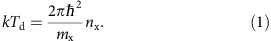Here me and mh are the electron and hole effective masses. For nx~1010 cm−2, and me=0.07, mh=0.15, mx=0.22 representative of GaAs CQW, we find Td~3 K. (Here and below all the masses are in units of the bare electron mass.) At such temperatures long-range spontaneous coherence of indirect excitons is observed5.

To explain why MoS2-based CQW would possess much higher Td than GaAs-based ones, we consider the characteristic length and energy scales in the problem, which are the exciton Bohr radius ax=ħ2ε1/μe2 and the Rydberg energy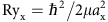defined in terms of reduced mass μ=memh/mx. For estimates we use the effective static dielectric constant ε1=4.9 of hBN. We also use the calculated value μ=0.25 (refs 7, 8) for MoS2. On the basis of the measurements9 done on a related compound MoSe2, this value should be accurate to within 20%. We find ax≈1.0 nm, much shorter than 15 nm in GaAs CQW. In turn, Ryx≈140 meV in the proposed device, about forty times larger than in GaAs.

Since it enters the denominator of equation (1), one may think that having larger mx is unfavourable for attaining higher Td. In fact, when nx can be controlled, the opposite is true. Indeed, equation (1) can be rewritten as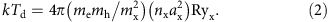The upper limit on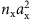is imposed by quantum dissociation of excitons that occurs when the ratio of the exciton size (the in-plane gyration radius) rx and the mean inter-exciton distance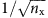reaches the critical value10,11,12 of about 0.3. In the case of our primary interest where interlayer centre-to-centre distance cax and me=mh=2μ, we estimate rx=2.4ax (Fig. 2), so that the corresponding Mott critical density nM is set by the condition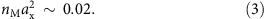Substituting this into equation (2), we obtain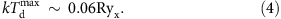Hence, the key to high Td is the enhanced value of Ryx. The exciton binding energy Eind≈0.6 Ryx (Fig. 2) does not pose further fundamental limitations. Actually, the conclusion that the theoretical maximum of Td is proportional to Ryx follows from dimensional analysis. If c~ax, me~mh and excitons are treated as an equilibrium Bose gas, then Ryx is the only relevant energy scale in the problem.

In reality, only quasi-equilibrium state is possible because of exciton recombination, and so limitations posed by the finite exciton lifetime τ must be discussed. Radiative recombination of indirect excitons requires interlayer tunnelling. The rate of this process can be made exponentially small by adjusting the barrier width. In GaAs CQW, τ can be routinely made in the range of microseconds and can be controllably varied over several orders of magnitude. The large τ enables creation of indirect exciton gases of high-density nx= with low optical excitation power P that does not cause overheating of either the lattice or the excitons. This is why for the task of achieving cold dense exciton gases, indirect excitons are superior to conventional bulk excitons or two-dimensional (2D) direct excitons (bound states of electrons and holes in the same layer).

In the proposed device, the interlayer tunnelling rate would decay exponentially with the number N of layers in the hBN spacer. For N=2, the tunnelling rate is comparable to that in the GaAs CQW4,5 (see Methods) indicating that long-life indirect excitons can be realized in MoS2/hBN structures. The tunnelling-limited lifetime can be enhanced by possible rotational misalignment of the MoS2 layers, which makes the excitons indirect not only in real but also in momentum space, similar to excitons in MoS2 multilayers9,13. Hence, sufficiently long τ may perhaps be achieved with a monolayer hBN spacer. Working with very small N, one has to worry about possibility of dielectric breakdown of the hBN spacer. To make the indirect exciton more energetically favourable than the direct one, a voltage equal or larger than (EdirEind)/e must be applied between the MoS2 layers. Assuming the direct exciton binding energy Edir of approximately8,14 0.5 eV, for N=2 hBN spacer, the required voltage is about 0.4 V, which is safely below the breakdown limit15.

A schematic phase diagram of a neutral electron–hole system in the proposed heterostructure is shown in Fig. 3. The solid line represents the Mott transition. It emanates from the T=0 critical point computed according to equation (3). At the transition there is a discontinuous jump in the degree of exciton ionization16. Above the Mott critical temperature TM the transition changes to a smooth crossover. Such a crossover has been studied experimentally in photo-excited single-well GaAs and InGaAs structures17,18. We assume that TM should be lower than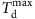and use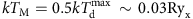in Fig. 3 for illustration. The quantum degeneracy line (equation 1) shown by the dashed-dotted line in Fig. 3 demarcates a crossover from classical to degenerate Bose gas of excitons. At n=nM this line extrapolates to the temperature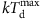(equation 4).

Whereas the Mott transition represents quantum dissociation of excitons, the thermal dissociation occurs above the Saha temperature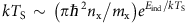, which is shown by the dashed line near the T axis in Fig. 3. This line marks a crossover from the exciton phase to a classical plasma. Since their binding energy is very large, indirect excitons in MoS2/hBN structure remain stable against the thermal dissociation well above the room temperature in a broad range of electron densities.

Formation of quantum degenerate Bose gas of long-lifetime repulsively interacting indirect excitons leads to local exciton superfluidity below Td. The superfluidity spreads over a macroscopic area at the Berezinskii–Kosterlitz–Thouless transition temperature19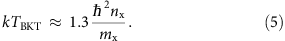Superfluid transport of neutral indirect excitons produces dissipationless charge currents in the opposite directions in the two layers2. To observe and utilize this effect, one can, for example, make separate contacts to each layer and form a closed circuit for the hole layer. The electric current in the electron layer will then be dissipationless. This is referred to as the counterflow superconductivity20.

The most intriguing conclusion we draw from Fig. 3 is that in the proposed MoS2/hBN structures degenerate Bose gas of indirect excitons can be realized at record-high temperatures.

## Discussion

In the remainder of this paper, we overview phenomena analogous to a coherent state of indirect excitons in other 2D systems. We choose not to survey systems where electrons and holes reside in the same layer, such as quantum wells in microcavities21. They are interesting in their own right but counterflow superconductivity therein is impossible.

First, evidence for broken-symmetry phases conceptually similar to condensates of indirect excitons have been reported in GaAs CQW22. These phases arise in the presence of a quantizing magnetic field at sub-Kelvin temperatures. Next, a number of broken-symmetry states, some of which are similar to exciton condensates, have been predicted to form in bilayer graphene23. The double-layer graphene (DLG) systems must be specially mentioned because a high-temperature coherent state in DLG was theoretically discussed recently. The fundamental obstacle to exciton condensation in DLG is that monolayer graphene is a zero-gap semimetal with a linear quasiparticle dispersion. Although in such a system phases of weakly bound excitons may exist, the corresponding critical temperature is extremely sensitive to the effective strength of the electron–hole attraction. Calculation of the latter requires accounting for screening of the long-range Coulomb interaction as well as short-range correlation effects, both of which are challenging problems. Theoretical estimates of the critical temperature in DLG range from hundreds of Kelvin24,25,26 to a few milli-Kelvin27. We wish to stress that there is no room for such an enormous uncertainty in our proposal based on MoS2, a semiconductor with a significant bandgap, modest dielectic constant and exceptionally stable exciton state. The dependence of the characteristic temperatures Td and TBKT on electron density is linear rather than exponential. All pertinent numerical factors are constrained by numerous prior studies of similar semiconducting systems. Therefore, the error in the estimated temperatures should be small for the system considered in our paper.

The scaling law of equation (4) elucidates a general principle for realizing high-temperature coherent states of excitons. The proposed design is an initial blueprint and is amenable to further optimization. For example, one28 or both of MoS2 layers can be substituted by a different transition metal dichalcogenide (TMD), such as WS2 or WSe2. The two MoS2 monolayers may be replaced by a single several-monolayer-thick TMD encapsulated by hBN in which indirect excitons would be composed of electrons and holes confined at the opposite sides of the TMD layer. The outlined principle for realizing high-temperature superfluidity can also be extended to layered materials other than TMD and hBN.

Besides providing a new platform for exploring fundamental quantum phenomena, indirect excitons in van der Waals structures can be also utilized for the development of optoelectronic circuits4. In such devices, in-plane potential landscapes for excitons are created and controlled by external electric fields that couple to the permanent dipole moment p=ec of the indirect excitons. The operation temperature for excitonic circuits in the van der Waals structures is expected to exceed by an order of magnitude the ~100 K record29 set by GaAs-based CQWs.

Finally, we note that Fig. 3 was constructed following the example of the Monte-Carlo simulations10,11,12 in which only exciton phases with two possible spin flavours were considered. In TMDs, spin and orbital degeneracies of indirect excitons may have a more intriguing structure. Such degeneracies can be controlled by strong spin–orbit coupling30, polarization of the excitation beam7,31 or many-body interactions32.

Experimental realization of superfluidity and counterflow superconductivity as well as excitonic circuits and spintronic/valleytronic devices in atomically thin heterostructures may have far-reaching implications for science and technology.

## Methods

### Interlayer tunnelling

The action S for tunnelling of quasiparticles across the hBN spacer can be estimated from the usual formula for the rectangular potential barrier of height Ub: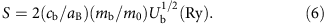Here cb=Nc1 is the tunnelling length, N is the number of hBN layers, c1=0.333 nm is the thickness of one hBN layer, aB=0.0529, nm is the hydrogen Bohr radius and mb is the effective carrier mass inside the barrier. If the chemical potential is in the middle of the hBN energy gap, we expect Ub≈3 eV. Assuming also mb≈0.5 in units of the bare electron mass, we obtain S≈5N, which is in agreement with S=4.6N deduced from the tunnelling conductance measured in graphene/hBN/graphene structures15. In comparison, in GaAs/AlGaAs CQW structures with mb≈0.35, Ub≈0.15 eV and db=4.0 nm we get S=9.4. Hence, for N=2 the tunnelling rate is comparable to that in the GaAs CQW4,5. More detailed estimates would have to include the trapezoidal shape of the barrier (Fig. 1b) and precise nature of the band alignment in the TMD/hBN structures. Nevertheless, it is clear that by a minor adjustment of N in the range from, say, 2 to 4, sufficiently long interlayer tunnelling lifetimes can be achieved for indirect excitons.

### Binding energy of indirect excitons

We model both the electron and the hole that compose an indirect exciton as 2D quantum particles confined in the mid-planes of two separate MoS2 layers. The Schrödinger equation for the relative motion reads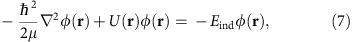where μ is the reduced mass. We model the potential U(r) of electron–hole Coulomb interaction using the continuum-medium electrostatics8. This simplified approach neglects frequency dependence of the dielectric functions of the materials involved. We approximate each of MoS2 layers as a uniaxial dielectric slab of thickness c2=0.312 nm with principal dielectric tensor components8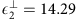and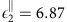in the directions perpendicular and parallel to the z axis, respectively. In turn, the hBN spacer is modelled as a slab of thickness Nc1, c1=0.333 nm, with the dielectric constants33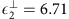and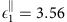. We define the anisotropy parameter κj and the effective dielectric constant εj of each material by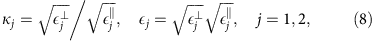so that κ2=1.44, ε2=9.91 (MoS2) and κ1=1.37, ε1=4.89 (hBN). The interaction potential can be found by solving the Poisson equation for this layered system, which is done by reducing it to a set of linear equations for the Fourier component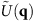and using the inverse Fourier transform: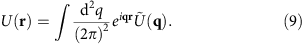By symmetry,depends only on the magnitude q=|q| of vector q not on its direction. After simple algebra, we arrive at the result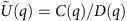, where functions C(q) and D(q) are given by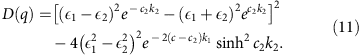Here we introduced the short-hand notations c=Nc1+c2 (the centre-to-centre distance) and kj=κjq (the z-direction wavenumber of the evanescent Fourier harmonics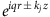in medium j). From numerical calculations using equations (9)–(11), , we found that potential U(r) is accurately approximated by the following analytical expression: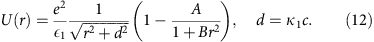With a suitable choice of coefficients 0<A<1 and B>0 this form produces asymptotically exact results for U(r) at both small and large r.

To solve equation (7), we discretized it on a real-space 2D grid (typically, 75 × 75). The resultant linear eigenvalue problem was diagonalized by standard numerical methods yielding the binding energy Eind(N) and the gyration radius rx(N) of indirect excitons. The latter is defined in terms of a normalized ground-state wavefunction φ(r) by means of the integral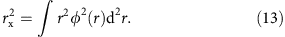The results are shown in Fig. 2. For N=2 we find rx=2.50 nm=2.43ax and Eind=87 meV≈0.6 Ryx. This binding energy is an order of magnitude larger than Eind=4–10 meV typical for excitons in GaAs/AlGaAs CQW structures29,34.

### Zero-temperature phases

It is instructive to complement the above discussion of the finite-T phase diagram (Fig. 3) with commenting on the T=0 phases. Such phases include electron–hole Fermi gas, exciton Bose gas and exciton solid. The approximate phase boundaries based on available Monte-Carlo calculations10,11,12,35,36 are shown in Fig. 4. Although those simulations were done for electron–hole bilayers in vacuum, an approximate correspondence with our dielectric environment can be achieved if we neglect the correction term in the parenthesis in equation (12). The mapping is then obtained by setting the distance between the layers to d=κ1c and including the effective dielectric function ε1 in the definition of the electron Bohr radius: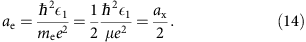On the horizontal axis in Fig. 4 we plot the dimensionless intralayer distance parameter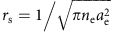, where ne is the total electron density. Solid phases form at large rs and d/ae. The exciton dipole solid crosses over to the interlocked Wigner crystals of electrons and holes as the interlayer distance becomes larger than the characteristic intralayer one, drsae.